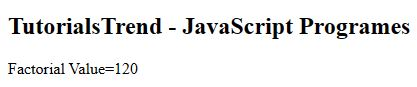﻿ JavaScript Factorial Program - TutorialsTrend
This Angular tutorial helps you get started with Angular quickly and effectively through many practical examples.
`

# JavaScript Factorial Program

#### Factorial Program

This program calculates the factorial of a given number. The factorial function says to multiply all the whole numbers from the chosen number down to one. In more mathematical terms, the factorial of a number (n!) is equal to n(n-1).

For example, if you want to calculate the factorial for four, you would write:

4! = 4 x 3 x 2 x 1 = 24.

Program

``````<!DOCTYPE html>
<html>
<title></title>
<body>
<h2>TutorialsTrend - JavaScript Programes</h2>
<script>

function factorial(num) {
if (num === 0) {
return 1;
} else {
return num * factorial(num - 1);
}
}
document.write("Factorial Value="+factorial(5)); // 120
</script>
</body>
</html>``````

OutputPrev Next